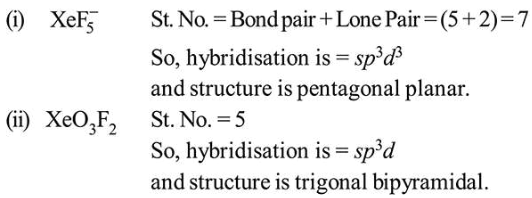# Solve the followingQuestion:

The shape/ structure of $\left[\mathrm{XeF}_{5}\right]^{-}$and $\mathrm{XeO}_{3} \mathrm{~F}_{2}$, respectively, are:

1. pentagonal planar and trigonal bipyramidal

2. octahedral and square pyramidal

3. trigonal bipyramidal and pentagonal planar

4. trigonal bipyramidal and trigonal bipyramidal

Correct Option: 1

Solution: